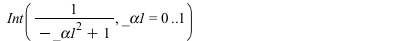## 11265 Reputation

6 years, 278 days

## The new ode...

 >
 > ################################ # Solution "by hand" # For simplicity, take a=0, b=1; ################################
 > restart;
 > de := diff(u(x),x\$4) = Heaviside(x - a)*u(x) - Heaviside(x - b)*u(x);(1)
 > a:=0;b:=1;(2)
 > dsolve(de, parametric); # wrong(3)
 > de1:=eval(de) assuming x<0; de2:=eval(de) assuming 0(4)
 > s1:=rhs(dsolve(de1)): s2:=rhs(dsolve(de2)): s3:=rhs(dsolve(de3)):
 > S:=piecewise(x<0, eval(s1,[_C1=c1,_C2=c2,_C3=c3,_C4=c4]), x<1, eval(s2,[_C1=c5,_C2=c6,_C3=c7,_C4=c8]), eval(s3,[_C1=c9,_C2=c10,_C3=c11,_C4=c12]));(5)
 > seq(seq(limit(diff(S,[x\$k]), x=p,left) - limit(diff(S,[x\$k]), x=p,right), k=0..3), p=[a,b]):
 > solve([%]):
 > SOL:=simplify(eval(S, %));(6)
 > indets(SOL, name);(7)
 > plot(eval(SOL,[c5=5,c6=6,c7=7,c8=8]), x=-1..2);>

## A way...

```{indices}(table(AA),'pairs');
```

## degree 5...

Your equation is polynomial of degree 5 in the unknown R, with huge symbolic coefficients.
Such equations usually do not have explicit solutions (see Abel–Ruffini theorem theorem and Galois theory).

## Corrupted worksheet...

The worksheet is corrupted, or contains some hidden fields.
If the .mw is exported as .mpl and then opened in a fresh session, it runs OK.

## maybe...

The documentation of SubgroupLattice says:

The algorithm used does not find any perfect subgroups of G, other than potentially G itself, its soluble residual, and the trivial group. If the soluble residual of G is of size at least 360, then it may contain other nontrivial perfect subgroups. These will not be found by the algorithm.

Maybe there are other omissions.

## Digits...

The denominator has many very small values ==> large roundoff errors.
Increasing Digits (e.g. Digits:=15) solves the problem.

## evalf...

If the matrix A has both exact and float entries, then it is obvious that some computations (e.g. the value of a discontinuous function) may return "unexpected" results.
Rank is such a discontinuous function. You have to accept this fact.
E.g. if A has two columns such that the first one is symbolic with at least an irrational entry,
and the second column is nonzero with float entries, the rank(A) is always 2.

 > restart;
 > v:=:
 > A:=;(1)
 > LinearAlgebra:-Rank(A);        # exact(2)
 > LinearAlgebra:-Rank(evalf(A)); # "approx" but probably "expected"(3)

## Just replace in param1,...,param4: ...

Just replace in param1,...,param4:  alpha*gamma=0.004;   with   gamma=0.004/alpha;
(or use algsubs).

- don't load packages which are not needed
- gamma is a Maple constant, don't use it as a variable
- linalg is deprecated; use LinearAlgebra instead.

## Int...

```convert(EllipticK(x), Int):
eval(%,x=1); value(%);```infinity

## size...

```with(GroupTheory):
g:=DrawSubgroupLattice(SmallGroup(200, 31), labels = ids, output=graph):
GraphTheory:-DrawGraph(g, size=[1200,800]);
```